### INTEGRATION OF TRIGONOMETRIC INTEGRALS

Recall the definitions of the trigonometric functions.

•••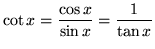•The following indefinite integrals involve all of these well-known trigonometric functions. Some of the following trigonometry identities may be needed.

• A.)• B.)• C.)so that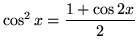• D.)so that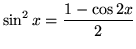• E.)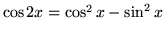• F.)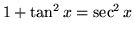so that• G.)so thatIt is assumed that you are familiar with the following rules of differentiation.

••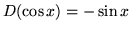•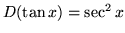•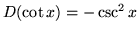•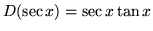•These lead directly to the following indefinite integrals.

• 1.)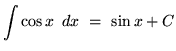• 2.)• 3.)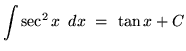• 4.)• 5.)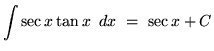• 6.)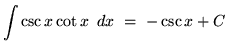The next four indefinite integrals result from trig identities and u-substitution.

• 7.)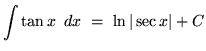• 8.)• 9.)• 10.)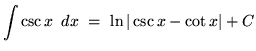We will assume knowledge of the following well-known, basic indefinite integral formulas :

•, where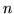is a constant•••, whereis a constant
•Most of the following problems are average. A few are challenging. Many use the method of u-substitution. Make careful and precise use of the differential notation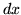and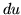and be careful when arithmetically and algebraically simplifying expressions.

• PROBLEM 1 : Integrate.

• PROBLEM 2 : Integrate.

• PROBLEM 3 : Integrate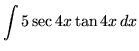.

• PROBLEM 4 : Integrate.

• PROBLEM 5 : Integrate.

• PROBLEM 6 : Integrate.

• PROBLEM 7 : Integrate.

• PROBLEM 8 : Integrate.

• PROBLEM 9 : Integrate.

• PROBLEM 10 : Integrate.

• PROBLEM 11 : Integrate.

• PROBLEM 12 : Integrate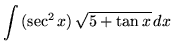.

• PROBLEM 13 : Integrate• PROBLEM 14 : Integrate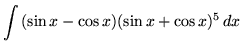.

• PROBLEM 15 : Integrate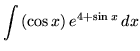.

• PROBLEM 16 : Integrate.

• PROBLEM 17 : Integrate.

• PROBLEM 18 : Integrate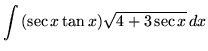.

• PROBLEM 19 : Integrate.

Some of the following problems require the method of integration by parts. That is,.

• PROBLEM 20 : Integrate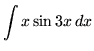.

• PROBLEM 21 : Integrate.

• PROBLEM 22 : Integrate.

• PROBLEM 23 : Integrate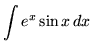.

• PROBLEM 24 : Integrate.

• PROBLEM 25 : Integrate.

• PROBLEM 26 : Integrate.

• PROBLEM 27 : Integrate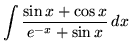.